Journal DetailsFormat
Journal
eISSN
1891-5469
First Published
01 Jan 2010
Publication timeframe
1 time per year
Languages
EnglishOpen Access

# Modeling and Simulation of Equivalent Circuits in Description of Biological Systems - A Fractional Calculus Approach

###### Received: 12 Dec 2011
Journal DetailsFormat
Journal
eISSN
1891-5469
First Published
01 Jan 2010
Publication timeframe
1 time per year
Languages
English

Using the fractional calculus approach, we present the Laplace analysis of an equivalent electrical circuit for a multilayered system, which includes distributed elements of the Cole model type. The Bode graphs are obtained from the numerical simulation of the corresponding transfer functions using arbitrary electrical parameters in order to illustrate the methodology. A numerical Laplace transform is used with respect to the simulation of the fractional differential equations. From the results shown in the analysis, we obtain the formula for the equivalent electrical circuit of a simple spectrum, such as that generated by a real sample of blood tissue, and the corresponding Nyquist diagrams. In addition to maintaining consistency in adjusted electrical parameters, the advantage of using fractional differential equations in the study of the impedance spectra is made clear in the analysis used to determine a compact formula for the equivalent electrical circuit, which includes the Cole model and a simple RC model as special cases.

#### Keywords

Introduction

According to Rigaud  who at the beginning of the 20th century began to study the structure of biological tissues based on their electrical properties, biological tissues are conductors and their resistance varies with frequency. The electrical property of any biological tissue depends on its intrinsic structure. In the case of human skin, the impedance can vary with the thickness and moisture content of the organ, the concentration and activity of sweat glands, injuries, age of subject and environmental factors such as temperature and humidity. Electrical impedance studies in biological systems, including human skin, generally, relate to direct measurements of impedance and phase angle as functions of frequency, voltage, or current applied [2, 3, 4, 5, 6]. In 1974, Burton  applied Bode analysis to measurements of impedance and phase angle of the skin. Through this method, a passive equivalent circuit can be used and considered as a "black box" to plot its impedance and phase angle versus frequency. The only necessary assumption is that the system consists entirely of linear passive elements [7, 8]. Although the resulting model is not necessarily unique , it describes the system with great precision in the range of frequencies studied.

Electrical impedance spectroscopy (EIS) has proven useful in the characterization of biomaterials by recording the behavior of their intrinsic properties while evaluating the bioelectrical response of the system as a sinusoidal excitation signal is applied . It has also been used to measure biological tissues in which the electrical impedance depends on water content and ionic conduction in the body. It should be noted that the terms “electrical impedance” and “resistance” are often used without distinction in literature . In terms of frequency, some researchers have reported that when the frequency is less than or equal to 10 kHz, the current does not cross the cell membrane, and thus the resistance obtained is relative only to the extracellular mass . This electrical conductivity is small in adipose tissue compared to fat-free tissue. The resistivity of components such as blood (150 Ωcm) or urine (40-200 Ωcm) is low, muscle (250-2000 Ωcm) and fat (2000-5000 Ωcm) are intermediate, and bone (10 000 Ωcm) is high. In the measurement of bioimpedance an electrical stimulus is applied and the response produced on a specific region of the body is analyzed. Usually, the stimulus is an alternating current signal of low amplitude intended to measure the electric field or potential difference generated between different parts of the tissue. The relationship between the data of the applied stimulus and the response obtained as a function of frequency provides the impedance spectrum of tissues studied .

Transfer function analysis is a mathematical approach to relate an input signal (or excitation) and the system response. The ratio formed by the pattern of the output and the input signal makes it possible to find the zeros and poles, respectively . A space state representation is a mathematical model of a physical system described by a set of inputs, outputs and state variables related by first order differential equations that are combined in a first order matrix differential equation. So as not to be affected by the number of inputs, outputs, and states, the variables are expressed as vectors, and algebraic equations are written in matrix form.

To analyze the behavior of the transfer function in the frequency domain, several graphical methods were used, such as Bode plots that provide a graphical representation of the magnitude and phase versus frequency of the transfer function . Nyquist plots are polar plots of impedance modulus and phase lag . One of the biggest drawbacks of working in polar coordinates is that the curve no longer retains its original shape when a modification to the system is made. For instance when the loop gain is altered, the magnitude-phase trace shifts vertically without distortion. However, when the properties of the phase are changed independently without affecting the gain, the magnitudephase trace is affected only in the horizontal direction.

The Cole impedance model was postulated in its final form by Kenneth Cole in 1940 . This impedance model is based on replacing the ideal capacitor in the Debye model  for a general element called constant phase element (CPE). The analysis of the Cole impedance model requires three things: (1) an equivalent circuit; (2) the development of the corresponding equations; and (3) a simulation which gives the complex impedance behavior.

The Cole model is represented by a series resistor (Rs), a capacitor (Cp) and a resistor in parallel (Rp), n is the order of the power that best fits the model obtained. Algebraically the circuit can be said to represent the total impedance as:

$ZT=RS+RP1+(jωRPCP)n.$$${{Z}_{T}}={{R}_{S}}+\frac{{{R}_{P}}}{1+{{\left( j\omega {{R}_{P}}{{C}_{P}} \right)}^{n}}}.$$

Using the Cole model parameters, this model corresponds tissue impedance values with the values of the components of circuit models .

Introduction to fractional calculus

Fractional calculus (FC) is nearly as old as the conventional calculus, but is not very popular within many scientific and engineering communities. The idea of FC seems to be quite a strange topic and very hard to explain, due to the fact that it is not related to some important physical meaning, such as velocity and acceleration, unlike commonly used differential calculus. For this reason it has long been of interest only to mathematicians. On the other hand, many physical phenomena have "intrinsic" fractional order descriptions and so FC is necessary in order to explain them. In many applications FC provides more accurate models of the physical systems than ordinary calculus does. Because of its success in description of anomalous diffusion [16, 17, 18], non-integer order calculus both in one and multidimensional space, has become an important tool in many areas of physics, mechanics, engineering, and bioengineering [19, 20, 21, 22, 23, 24, 25, 26]. Fundamental physical considerations in favor of the use of models based on derivatives of non-integer order are given in Caputo & Mainardi and Westerlund [27-28]. Fractional derivatives provide an excellent instrument for the description of memory and hereditary properties of various materials and processes. This is the main advantage of FC in comparison with the classical integer-order models, in which such effects are in fact neglected. The other large field requiring the use of FC is the theory of fractals . The development of the theory of fractals has opened further perspective for the theory of fractional derivatives, especially in modeling dynamical processes in self-similar and porous structures.

To analyze the dynamical behavior of a fractional system it is necessary to use an appropriate definition of fractional derivative. In fact, the definitions of the fractional order derivative are not unique and there exist several definitions, including Grünwald-Letnikov, Riemann-Liouville, Weyl, Riesz, and the Caputo representation. In the Caputo case, the derivative of a constant is zero and we can properly define the initial conditions for the fractional differential equations so that they can be handled analogously to the classical integer case. Caputo derivative implies a memory effect by means of a convolution between the integer order derivative and a power of time [30, 31, , 32, 33]. For these reasons, in this paper we prefer to use the Caputo fractional derivative defined by:

$Dγf(t)=1Γ(n−γ)∫0tf(n)(τ)(t−τ)γ+1−ndτ,$$${{D}^{\gamma }}f\left( t \right)=\frac{1}{\Gamma \left( n-\gamma \right)}\int\limits_{0}^{t}{\frac{{{f}^{\left( n \right)}}\left( \tau \right)}{{{\left( t-\tau \right)}^{\gamma +1-n}}}d\tau ,}$$

where n-1<γ<n, and f(n)(τ) represents the derivative of order n, real function evaluated in t. Working with this definition is important because of its ability to be implemented numerically . Another very important feature in the form of Caputo fractional derivative is that its Laplace transform is:

$L{Dγf(t)}=sγF(s)−∑k=0n−1f(k)(0)sγ−k−l$$$L\left\{ {{D}^{\gamma }}f\left( t \right) \right\}={{s}^{\gamma }}F\left( s \right)-\sum\limits_{k=0}^{n-1}{{{f}^{\left( k \right)}}\left( 0 \right){{s}^{\gamma -k-l}}}$$

From equation (3), using the initial conditions f(k)(0) where k is integer we can see that the representation of the Caputo derivative in Laplace domain reduces to:

$L{Dγf(t)}=sγF(s)$$$L\left\{ {{D}^{\gamma }}f\left( t \right) \right\}={{s}^{\gamma }}F\left( s \right)$$

This is consistent with the usual definition of the Laplace transform when γ is an integer. In general, a fractional order differential equation has the form

$∑k=0nakDγkf(t)=g(t),$$$\sum\limits_{k=0}^{n}{{{a}_{k}}{{D}^{{{\gamma }_{k}}}}f\left( t \right)}=g\left( t \right),$$

where ɣk>ɣk-1 and ak are any real numbers and g(t) is the source of a dynamic system. The inverse transform 0<ɣ≤1 requires the introduction of a special function, the Mittag-Leffler function, where Γ is the gamma function defined as

$Ea,b(t)=∑m=0∞tmΓ(am+b), (a,b>0),$$${{E}_{a,b}}\left( t \right)=\sum\limits_{m=0}^{\infty }{\frac{{{t}^{m}}}{\Gamma \left( am+b \right)}},\ \ \left( a,b>0 \right),$$

From (5) if a=1, b=1, then we obtain the expression E1,1(t)=et. Therefore, the Mittag-Leffler function includes the exponential as a special case.

Numerical Laplace transform

The Laplace transform is a useful tool for analyzing linear systems because it simplifies the problem of dealing with differential equations in the time domain by converting them into algebraic equations within the frequency domain. The numerical Laplace transform (NLT) is essentially a modified discrete Fourier transform (DFT) through a windowing function (Gibbs phenomenon) and a stability factor (aliasing) . Development of the NLT and its application to the analysis of systems has been well documented over the past 40 years [36-37]. However, its use has traditionally limited the analysis of problems where the result can be expressed in terms of simple functions that allow the use of tables of Laplace transforms . When using discrete techniques in the frequency domain computation time becomes an important factor since it requires a certain amount of time to transform the data from the frequency domain to the time domain or vice versa. However, by using the fast Fourier transform (FFT) the time necessary for computation is greatly reduced and as a result the techniques of analysis in the frequency domain become an attractive option.

Sheng investigated the validity of applying numerical inverse Laplace transform algorithms in fractional calculus . In a paper of Gómez an overview of a methodology based on the NLT and its application to analysis of a three physical system from the point of view of FC is discussed .

In this work for the multilayer biological system, we propose an analysis of an electrical circuit equivalent consisting of epidermis, dermis and the subcutaneous tissue. The response of the Bode diagram is interpreted and compared with the response to the modeling of integer order (using transfer function analysis) and fractional order (obtained through NLT techniques). The modeling of electrical impedance spectra applied to the study of experimental data of blood is made using the Cole model electrical impedance equations. The Nyquist diagrams (frequency response) are also compared for both integer and fractional order model responses.

Methodology
Complex electrical circuit in the Laplace space and its application to distributed elements

In order to apply the general theory to the skin type system, we propose an equivalent electrical circuit for each component of the skin. Fig. 1 shows the schematic diagram to describe the proposed model of the skin, where Ra and Rb are the contact resistances of the electrodes, De represents the equivalent circuit of the dermis (R1, C1, R2, C2, R3, C3), G corresponding to the fat equivalent circuit (R4, C4, R5, C5, R7, C7), and finally, the circuit for the muscle (R6, C6). Dividing the equivalent circuit in the corresponding layers: dermis, subcutaneous tissue (fat) and muscle, we will consider it as an electrical network with the following parameter values, which in Fig. 1 appear as per unit values , and correspond to Ra=Rb=0.08, R1=R2=R3=0.4, C1=C2=C3=0.2, R4=R5=R7=0.7, C4=C5=C7=0.3, R6=0.5, C6=0.5.

It is known that in biological systems, the module of the impedance is inversely dependent on frequency and does not show phenomena of conversion of electrical energy to magnetic energy [41-42]. Therefore, it is considered appropriate to model a biological system as only having capacitive and resistive behavior. Fig. 2 shows the circuit equivalent to the first layer of the system. Applying Kirchhoff's Laws the following representation of state equations is obtained:

$x˙=Ax+Bu$$$\dot{x}=Ax+Bu$$$y=Cx+Du$$$y=Cx+Du$$

where $A=−1C1(1R1+1Ra+Rb), B=1C1(Ra+Rb),$$\text A=-\frac{1}{{{C}_{1}}}\left( \frac{1}{{{R}_{1}}}+\frac{1}{{{R}_{a}}+{{R}_{b}}} \right),\ \ \ \ \ B=\frac{1}{{{C}_{1}}\left( {{R}_{a}}+{{R}_{b}} \right)},$

is called the state vector, y is the output vector and u is a vector of inputs (or control). A is the matrix of states, B is the input matrix, x is a column vector representing the voltage on the capacitor, C is the matrix output (voltage on the capacitor that models the dermis), C1=1 and D is the feedforward matrix (for this model D=0). The values of the parameters of the circuit in Fig. 2 correspond to Ra=Rb=0.08, C1=0.2 and R1=0.4. The transfer function of the first layer is

$H(s)=31.25s+43.75.$$$H\left( s \right)=\frac{31.25}{s+43.75}.$$

Fig. 3 shows the corresponding Bode plot.

$A=[(Ra+Rb)C1RaC2RbC3−C4C2+C4C4−C4C4C3+C4]−1[−1+Ra+RbR1−RaR2−RbR31C4−(1R2+1R4)−1R41R4−1R4−(1R3+1R4)] B=[(1Ra+Rb)C1 0 0]T C=[0,0,1] and D=.$\begin{align}& A={{\left[ \begin{matrix}\left( {{R}_{a}}+{{R}_{b}} \right){{C}_{1}} & {{R}_{a}}{{C}_{2}} & {{R}_{b}}{{C}_{3}} \\-{{C}_{4}} & {{C}_{2}}+{{C}_{4}} & {{C}_{4}} \\-{{C}_{4}} & {{C}_{4}} & {{C}_{3}}+{{C}_{4}} \\\end{matrix} \right]}^{-1}}\left[ \begin{matrix}-1+\frac{{{R}_{a}}+{{R}_{b}}}{{{R}_{1}}} & -\frac{{{R}_{a}}}{{{R}_{2}}} & -\frac{{{R}_{b}}}{{{R}_{3}}} \\\frac{1}{{{C}_{4}}} & -\left( \frac{1}{{{R}_{2}}}+\frac{1}{{{R}_{4}}} \right) & -\frac{1}{{{R}_{4}}} \\\frac{1}{{{R}_{4}}} & -\frac{1}{{{R}_{4}}} & -\left( \frac{1}{{{R}_{3}}}+\frac{1}{{{R}_{4}}} \right) \\\end{matrix} \right] \\ & \ \ \ \ \ \ \ \,\ B={{\left[ \left( \frac{1}{{{R}_{a}}+{{R}_{b}}} \right){{C}_{1}}\ 0\ 0 \right]}^{T}}\ C=\left[ 0,0,1 \right]\ \ \text{and}\ \ D=\left[ 0 \right]. \\ \end{align}$A=Ra+RbC1RaC2RbC300−C4C2+C4C4−C50−C4C4C3+C40−C7−C6C6C6C5+C6C6C6−C6−C6−C6−C6+C7−1−1+Ra+RbR1−RaR2−RbR3001C4−1R2+1R4−1R41R501R4−1R4−1R3+1R401R71R6−1R6−1R6−1R5+1R6−1R6−1R61R61R61R6−1R6+1R4B=1Ra+RbC10000TC=0,0,0,0,1andD=0.$\begin{align}& A={{\left[ \begin{matrix}\left( {{R}_{a}}+{{R}_{b}} \right){{C}_{1}} & {{R}_{a}}{{C}_{2}} & {{R}_{b}}{{C}_{3}} & 0 & 0 \\-{{C}_{4}} & {{C}_{2}}+{{C}_{4}} & {{C}_{4}} & -{{C}_{5}} & 0 \\-{{C}_{4}} & {{C}_{4}} & {{C}_{3}}+{{C}_{4}} & 0 & -{{C}_{7}} \\-{{C}_{6}} & {{C}_{6}} & {{C}_{6}} & {{C}_{5}}+{{C}_{6}} & {{C}_{6}} \\{{C}_{6}} & -{{C}_{6}} & -{{C}_{6}} & -{{C}_{6}} & -\left( {{C}_{6}}+{{C}_{7}} \right) \\\end{matrix} \right]}^{-1}}\left[ \begin{matrix}-1+\frac{{{R}_{a}}+{{R}_{b}}}{{{R}_{1}}} & -\frac{{{R}_{a}}}{{{R}_{2}}} & -\frac{{{R}_{b}}}{{{R}_{3}}} & 0 & 0 \\\frac{1}{{{C}_{4}}} & -\left( \frac{1}{{{R}_{2}}}+\frac{1}{{{R}_{4}}} \right) & -\frac{1}{{{R}_{4}}} & \frac{1}{{{R}_{5}}} & 0 \\\frac{1}{{{R}_{4}}} & -\frac{1}{{{R}_{4}}} & -\left( \frac{1}{{{R}_{3}}}+\frac{1}{{{R}_{4}}} \right) & 0 & \frac{1}{{{R}_{7}}} \\\frac{1}{{{R}_{6}}} & -\frac{1}{{{R}_{6}}} & -\frac{1}{{{R}_{6}}} & -\left( \frac{1}{{{R}_{5}}}+\frac{1}{{{R}_{6}}} \right) & -\frac{1}{{{R}_{6}}} \\-\frac{1}{{{R}_{6}}} & \frac{1}{{{R}_{6}}} & \frac{1}{{{R}_{6}}} & \frac{1}{{{R}_{6}}} & -\left( \frac{1}{{{R}_{6}}}+\frac{1}{{{R}_{4}}} \right) \\\end{matrix} \right] \\ & B={{\left[ \left( \frac{1}{{{R}_{a}}+{{R}_{b}}} \right){{C}_{1}} 0 0 0 0 \right]}^{\text{T}}} C=\left[ 0,0,0,0,1 \right] \text{and} D=\left[ 0 \right]. \\ \end{align}

The circuit parameters are chosen by establishing that the array is not indeterminate. If any row of the matrix is zero, a branch of the circuit (Fig. 1) has been removed.

Following the above procedure, we next obtained the equations of state considering only the first two layers and finally for all three layers and their corresponding Bode plots, showing the effect of each layer. In equations (10) and (11) the matrices and vectors for A, B, C and D, for the first two layers and all three layers, respectively, are shown. The matrix representation is a two-dimensional table of numbers consisting of abstract quantities that can be added and multiplied. The equations (10) and (11) describe the system of linear equations and keep track of the coefficients of linear recording data that depend on various parameters.

The transfer functions of the second and third layers are shown in equations (12) and (13), respectively.

$H(s)=380.5s+5528s3+55.03s2+862.6s+3983.$$$H\left( s \right)=\frac{380.5s+5528}{{{s}^{3}}+55.03{{s}^{2}}+862.6s+3983}.$$$H(s)=103.9s3+3946s2+4.916s+199900s5+66.98s4+1562s3+16160s2+73340s+109400$$$H\left( s \right)=\frac{103.9{{s}^{3}}+3946{{s}^{2}}+4.916s+199900}{{{s}^{5}}+66.98{{s}^{4}}+1562{{s}^{3}}+16160{{s}^{2}}+73340s+109400}$$

In each case, (12) and (13), the input passes through the circuit and reflects the voltage of the capacitor under study. Thus, the transfer function is related to a different output in each case for the same input. Fig. 4 shows the Bode diagram corresponding to the second and third layer.

Fractional analysis of the general model in the Laplace space and general model for the equivalent electrical circuit

Applying the formalism of fractional calculus to the circuit of Fig. 2 gives the fractional Laplace transform. For this purpose, the fractional time derivative operator of order γ can be written as follows

$dγdtγ, 0<γ≤1,$$$\frac{{{d}^{\gamma }}}{d{{t}^{\gamma }}},\ \ \ \ 0<\gamma \le 1,$$

where γ is an arbitrary parameter, which representing the order of the derivative and in the case γ=1 becomes the usual derivative operator d/dt.

Expression (14) is not an ordinary (usual) time derivative operator because the dimensionality is s. In order to retain consistency of units (dimensionality) we introduce a new parameter, σ, as follows

$ddt→1σ1−γdγdtγ, n-1<γ≤n,$$$\frac{d}{dt}\to \frac{1}{{{\sigma }^{1-\gamma }}}\frac{{{d}^{\gamma }}}{d{{t}^{\gamma }}},\ \ \ \ \ n\text{-}1<\gamma \le n,$$

which is true if the parameter σ has dimensions of seconds, [σ] = s, and n is an integer .

Expression (15) is a time derivative in the usual sense, because its dimension is s-1. The parameter σ represents the fractional time components in the system (such components change the time constant of the system). This non-local time is called the cosmic time in the literature . Another physical and geometrical interpretation of the fractional operators is given in Moshre-Torbati and Hammond .

The fractional differential equation for the circuit of Fig. 2 is

$dVcdt=[−σ1−γC1(Ra+Rb+R1(R1)(Ra+Rb))][Vc]+[σ1−γC1(Ra+Rb)]U,$$$\frac{d{{V}_{c}}}{dt}=\left[ -\frac{{{\sigma }^{1-\gamma }}}{{{C}_{1}}}\left( \frac{{{R}_{a}}+{{R}_{b}}+{{R}_{1}}}{\left( {{R}_{1}} \right)\left( {{R}_{a}}+{{R}_{b}} \right)} \right) \right]\left[ {{V}_{c}} \right]+\left[ \frac{{{\sigma }^{1-\gamma }}}{{{C}_{1}}\left( {{R}_{a}}+{{R}_{b}} \right)} \right]U,$$

the parameter values appear as per unit values  and correspond to Ra=Rb=0.08, C1=0.2 and R1=0.4.

The Laplace transform for (16) is

$V(s)=Uσ1−γ[sγ+σ1−γC1R1+σ1−γC1(Ra+Rb)][C1(Ra+Rb)].$$$V\left( s \right)=\frac{U{{\sigma }^{1-\gamma }}}{\left[ {{s}^{\gamma }}+\frac{{{\sigma }^{1-\gamma }}}{{{C}_{1}}{{R}_{1}}}+\frac{{{\sigma }^{1-\gamma }}}{{{C}_{1}}\left( {{R}_{a}}+{{R}_{b}} \right)} \right]\left[ {{C}_{1}}\left( {{R}_{a}}+{{R}_{b}} \right) \right]}.$$

Applying NLT algorithm to the frequency response obtained is shown in Fig. 5. As a case study the values of γ=0.95 and γ=0.98 were used.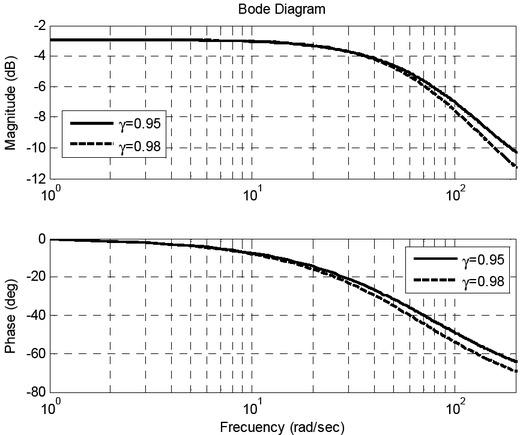Bode diagram corresponding to the first layer, fractional exponent γ=0.95

Fig. 6 shows the comparison for the Bode diagram for the integer order and the fractional order. The second and third layers had similar results.

Modeling of experimental data of blood tissue

Three samples were obtained from the blood transfusion center in Leon, Guanajuato.

All of the samples were clinically examined according to the blood transfusion protocol, to confirm they were free of contagious diseases such as HIV, hepatitis, and brucellosis. Cell populations were monitored through a counting study using a flow cytometer type Coulter. This was done so that cell mortality did not affect the results. To obtain the electrical impedance spectra a Solartron® 1260 was used. The fidelity of the frequency sweep for these tests was important since it shows the characteristic spectrum of the sample, which is necessary for a comparison with the electrical parameters of an equivalent circuit. The frequency range used was from 10Hz to 100kHz. The samples were placed in Bayer® strips. A frequency sweep with constant voltage amplitude of 25 mV was applied through two electrodes integrated in the Bayer® container. All test points were coated with silver and therefore had negligible contributions to polarization, and thus did not need to be compensated for. Once the measurements were represented in a Nyquist diagram, we obtained representations of equivalent electrical circuits via the software ZView®. The method of "instant adjustment" was used to fit the data to predefined circuit models. Fig. 7 shows the equivalent network for the measurement of erythrocytes, leukocytes and plasma.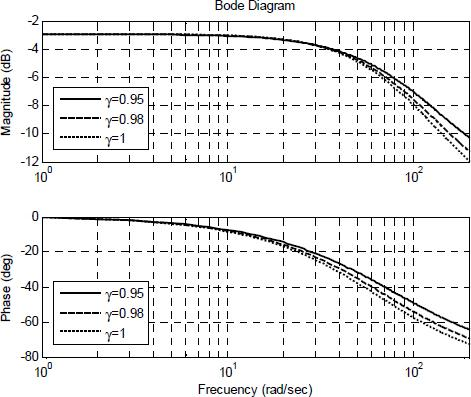Bode diagram comparison corresponding to the first layer, integer order and fractional exponent γ=0.95 and γ=0.98.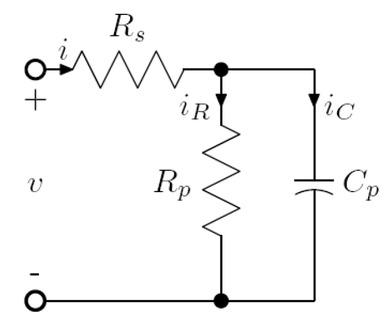Equivalent electrical circuit for data of blood tissue, erythrocytes, leukocytes and plasma.

Table 1 shows the values for the erythrocytes, leukocytes and plasma, for the samples 1, 2, and 3, respectively. The units of resistance are in ohms and the capacitors are farads.

Values for the erythrocytes, leukocytes and plasma.

ErythrocytesLeukocytesPlasma
Rs627.2444.4431.4
673.7475.6496.5
715.9460.1460.8
Cp2.2081×10-82.43×10-82.4055×10-8
2.2900×10-82.188×10-82.1517×10-8
2.2575×10-82.2336×10-82.4114×10-8
Rp149970358000261960
120710341550273750
136930332340252250

To determine the equivalent equation, the impedance is found by the following formula in the complex frequency domain:

$Z(s)=V(s)I(s)$$$Z\left( s \right)=\frac{V\left( s \right)}{I\left( s \right)}$$

Applying Kirchhoff laws to the circuit of Fig. 7, we have:

$V=Rsi+Vcp$$$V={{R}_{s}}i+V{{c}_{p}}$$$i=iR+ic$$$i={{i}_{R}}+{{i}_{c}}$$

Before applying the Laplace transform of (19) and (20) the following considerations must be made

$iR=VcpRp$$${{i}_{R}}=\frac{V{{c}_{p}}}{{{R}_{p}}}$$$ic=CpdVcpdt$$${{i}_{c}}={{C}_{p}}\frac{dV{{c}_{p}}}{dt}$$

Working with our definition of the fractional derivative , (21) and (22) become:

$iR=VcpRp$$${{i}_{R}}=\frac{V{{c}_{p}}}{{{R}_{p}}}$$$ic=CpdγVcpdtγ$$${{i}_{c}}={{C}_{p}}\frac{{{d}^{\gamma }}V{{c}_{p}}}{d{{t}^{\gamma }}}$$

Substituting (23) and (24) into (20), we obtain:

$i=VcpRp+CpdγVcpdtγ$$$i=\frac{V{{c}_{p}}}{{{R}_{p}}}+{{C}_{p}}\frac{{{d}^{\gamma }}V{{c}_{p}}}{d{{t}^{\gamma }}}$$

Applying the Laplace transform to (19) and (25):

$V(s)=RsI(s)+Vcp(s)$$$V\left( s \right)={{R}_{s}}I\left( s \right)+V{{c}_{p}}\left( s \right)$$$I(s)=Vcp(s)Rp+Cpσ1−γsγVcp(s)$$$I\left( s \right)=\frac{V{{c}_{p}}\left( s \right)}{{{R}_{p}}}+\frac{{{C}_{p}}}{{{\sigma }^{1-\gamma }}}{{s}^{\gamma }}V{{c}_{p}}\left( s \right)$$

Finally from (26) and (27) we have:

$Z(s)=Rs+Rp1+RpCpσ1−γsγ$$$Z\left( s \right)={{R}_{s}}+\frac{{{R}_{p}}}{1+\frac{{{R}_{p}}{{C}_{p}}}{{{\sigma }^{1-\gamma }}}{{s}^{\gamma }}}$$

If, s=(jω), we have:

$Zjw=Rs+Rp1+RpCpσ1−γjωγ$$$Z\left( jw \right)={{R}_{s}}+\frac{{{R}_{p}}}{1+\frac{{{R}_{p}}{{C}_{p}}}{{{\sigma }^{1-\gamma }}}{{\left( j\omega \right)}^{\gamma }}}$$

Equation (29) is the result of the fractional temporal operator in the equation for the RC equivalent circuit; this general formula includes an arbitrary constant, σ, which can be considered its own bioelectric parameter. In the particular case of σ=RPCP, the model is reduced to the Cole model. On the other hand, if we make γ=1, we obtain an ideal RC circuit. Figs. 8, 9 and 10 show the experimental results represented in the form of a Nyquist plot (lines with circles), the plot obtained from the solution of the circuit of Fig. 7 ( line with +), and the plot obtained by applying the definition of fractional calculus (line with *). Nyquist diagrams are sensitive to changes in the spectra of similar samples but even in this representation we can see that the measurements on the same type of cell (erythrocytes) present a similar behavior: semi-circles with a diameter of around 150 kΩ. The description of the spectra for both leukocytes and plasma leads to a similar argument about the reproducibility of the experiment.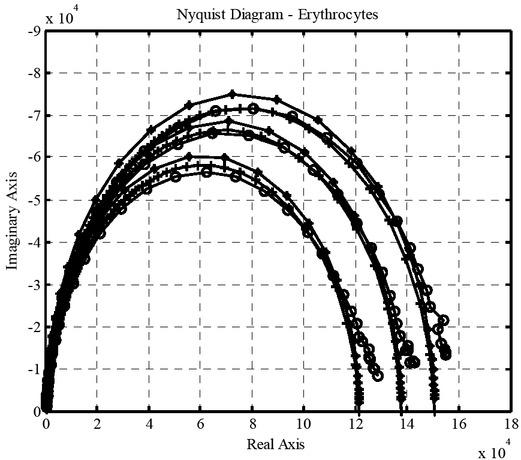Nyquist diagram for erythrocytes showing reactance as a function of resistance.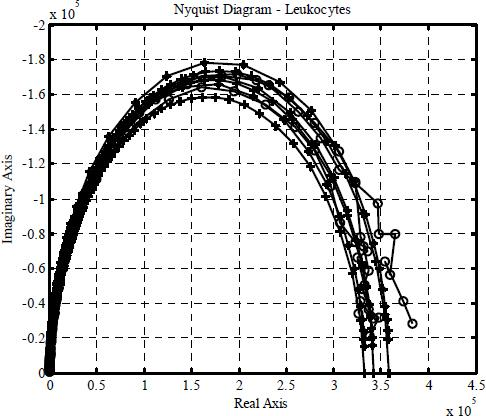Nyquist diagram for leukocytes showing reactance as a function of resistance.
Results

In this work we have analyzed in detail the transfer function of a multilayer system. Fig. 3 shows the Bode plot to the magnitude (top graph) and phase (bottom graph). In the first graph of Fig. 3, we see by increasing frequency, from the cutoff frequency (30 rad/s), the magnitude decreases at a rate of 20 dB/decade, while for frequencies below the cutoff magnitude it is almost constant.

This means that the current through the dermis is higher with decreasing frequency and is lower as the frequency increases. This means that the current through the dermis increases for higher frequencies. The attenuation in the first layer is solely due to the resistance of the electrodes. The current flowing through these resistors at low frequencies causes a Joule effect, which raises the temperature and therefore the kinetic energy of the molecules that make up the layer . As the frequency increases, other phenomena occur, such as displacement currents, ionization, polarization, and so on. Concerning phase, we have shown that by increasing frequency the displacement current and polarization are also increased (Fig. 3, bottom graph). This causes a decrease in phase 0° to -90° for very high frequencies.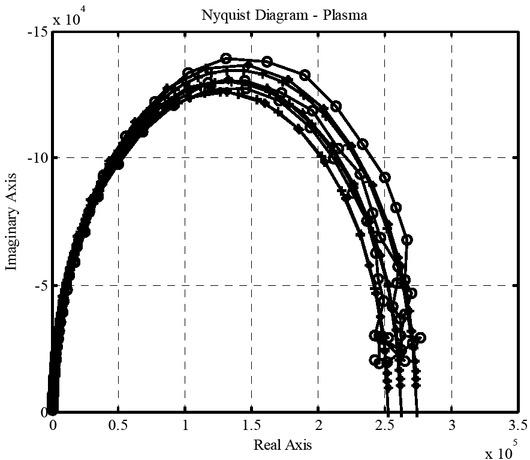Nyquist diagram for plasma showing reactance as a function of resistance.

There is not a significant decrease in the rate of change of the magnitude on the frequency, as can be seen from equations (9) and (10), where the difference in poles and zeros is two. By increasing the number of layers, the output voltage in the layer farthest from the source is attenuated at a faster rate due to the resistance of the previous layers and the increase in frequency. Thus the current in the deeper layers of the skin decreases more rapidly with increasing frequency. Fig. 4 shows that adding layers to the model changes the cutoff frequency – hence the shift in the phase – and the magnitude decreases from 20 dB/decade to 40 dB/decade. For the range of frequency 10 to 100 rad/s a shift in the magnitude and phase is shown, which implies that the proposed circuit better defines these frequencies.

In the first graph of Fig. 5, we see that by increasing frequency from the cutoff frequency (10 rad/s), the magnitude decreases at a rate of 6 dB/decade, while for frequencies below the cutoff magnitude it is almost constant. This means that the current through the dermis is very high with decreasing frequency and is very low when the frequency increases. The current flowing through these resistors at low frequencies causes a fractional Joule effect (behavior between a system conservative and dissipative), which raises the temperature and therefore the kinetic energy of the molecules that make up the layer. Concerning the phase Fig. 5 (bottom graph), we have that by increasing frequency the displacement current and polarization are very much increased.

With respect to the data of blood tissue, the families of curves of erythrocytes, leukocytes, and blood plasma are found to have similar parameters (R and C). This capability can be exploited experimentally for characterization, study, and research of blood tissue. In the literature it is common to characterize based on leastsquares fit of equivalent electrical circuit models on experimental data, including Cole models. From the description of the fractional differential equation models it can be noted that the representation of Cole models is derived as a particular solution to the RC circuit under fractional calculus. The simulations obtained from the fractional representation provide a better description than those obtained by the equations of integer order. The table 2 shows the exponent of the fractional differential equation that best fits the data for erythrocytes, leukocytes and plasma.

Exponent of the fractional differential equation that best fits the data

ErythrocytesLeukocytesPlasma
γ0.970.980.995
0.9750.990.99
0.980.970.9997
Discussion and Conclusions

Fractional calculus has been used successfully to modify many existing models of physical processes. The representation of equivalent models in integer order derivatives provided a good approximation of the bioelectric response of the model. However, with the formal introduction of fractional calculus to the study of fractional derivative systems have a better approximation of this response. This is due in part to the nature of systems described by fractional calculus.

On the basis of Cole’s proposal to add a degree of extra freedom to solve the RC circuit for characterization purposes and to improve the correlation in the adjustment to experimental data, we have developed analytical arguments to derive this result based on integration in weighted individual relaxation processes. However, the distributions of relaxation times involve complex functions and are difficult to measure. This study has shown a pattern in which the Cole type behavior appears as a result of competition between a capacitive and resistive behavior within the sample, characterized by the fractional order derivative of the applied voltage. The new generalized model includes the Cole model and the simple RC circuit as particular cases.

The models for EIS are assumed to be linear in their first approximation. The electrical parameters only take a nonlinear behavior in the case of tissue damage due to the excessive power in the supply or in the presence of physical and chemical reactions in the sample induced by the input current (exothermic processes or release of electrons). The electrical conduction, even for alternating current, has impedance that depends on the temperature, which turns the frequency response into a function of temperature.

#### Exponent of the fractional differential equation that best fits the data

ErythrocytesLeukocytesPlasma
γ0.970.980.995
0.9750.990.99
0.980.970.9997

#### Values for the erythrocytes, leukocytes and plasma.

ErythrocytesLeukocytesPlasma
Rs627.2444.4431.4
673.7475.6496.5
715.9460.1460.8
Cp2.2081×10-82.43×10-82.4055×10-8
2.2900×10-82.188×10-82.1517×10-8
2.2575×10-82.2336×10-82.4114×10-8
Rp149970358000261960
120710341550273750
136930332340252250

Rigaud B., Hamzaoui L., Chauveau N., Granie M., Scotto Di Rinaldi JP, and Morucci JP. Tissue characterization by impedance: a multifrequency approach. Physiol. Meas. 1994; 15: A13-A20. http://dx.doi.org/10.1088/0967-3334/15/2A/002808703510.1088/0967-3334/15/2A/002RigaudB.HamzaouiL.ChauveauN.GranieM.ScottoDi Rinaldi JPMorucciJPTissue characterization by impedance: a multifrequency approachPhysiol. Meas199415A13A20http://dx.doi.org/10.1088/0967-3334/15/2A/002Search in Google Scholar

Edelberg R. Biophysical Properties of the Skin, Elden HR, Editor. John Wiley & Sons, New York. 1971; 513-550.EdelbergRBiophysical Properties of the SkinEldenHRJohn Wiley & SonsNew York1971513550Search in Google Scholar

Cole KS. Cold Spring Harbor Symp. Quant. Biol. 1933;1: 107. http://dx.doi.org/10.1101/SQB.1933.001.01.014ColeKSCold Spring Harbor SympQuant. Biol19331107http://dx.doi.org/10.1101/SQB.1933.001.01.01410.1101/SQB.1933.001.01.014Search in Google Scholar

Hozawa S. Arch. Phys. 1928;219:111.Hozawa S. Arch. Phys.192821911110.1007/BF01723405Search in Google Scholar

Plutchik R., and Hirsch HR. Skin Impedance and Phase Angle as a Function of Frequency and Current. Science, 1963;141:919-927. http://dx.doi.org/10.1126/science.141.3584.927PlutchikR.HirschHRSkin Impedance and Phase Angle as a Function of Frequency and CurrentScience1963141919927http://dx.doi.org/10.1126/science.141.3584.92710.1126/science.141.3584.92714043339Search in Google Scholar

Stephens WGS. Med. Electron. Biol. Eng. 1963;1:384-389. http://dx.doi.org/10.1007/BF02474422StephensWGSMed. Electron. Biol. Eng19631384389http://dx.doi.org/10.1007/BF0247442210.1007/BF02474422Search in Google Scholar

Burton CE, David RM, Portnoy WM, and Akers LA. The application of Bode analysis to skin impedance. Psychophysiology. 1974;11(4):517-25. http://dx.doi.org/10.1111/j.1469-8986.1974.tb00581.x485465510.1111/j.1469-8986.1974.tb00581.xBurtonCEDavidRMPortnoyWMAkersLAThe application of Bode analysis to skin impedancePsychophysiology197411451725http://dx.doi.org/10.1111/j.1469-8986.1974.tb00581.x4854655Search in Google Scholar

Van Valkenburg ME. Network Analysis, 3rd ed. Prentice-Hall, Englewood Cliffs, N.J, 1974.VanValkenburg MENetwork Analysis, 3rd edPrentice-HallEnglewood Cliffs, N.J1974Search in Google Scholar

Sosa M., Bernal-Alvarado J.. Magnetic field influence on electrical properties of human blood measured by impedance spectroscopy. Bioelectromagnetics. 2005;26(7):564–570. http://dx.doi.org/10.1002/bem.201321614278010.1002/bem.20132SosaM.Bernal-AlvaradoJ.Magnetic field influence on electrical properties of human blood measured by impedance spectroscopyBioelectromagnetics2005267564570http://dx.doi.org/10.1002/bem.2013216142780Search in Google Scholar

Fredix HM., Saris HM., Soeters PB., Wouters FM., and Kester DM. Estimation of body composition by bioelectrical impedance in cancer patients. European Journal of Clinical Nutrition. McMillan Press. 2009;44:749-752.FredixHM.SarisHM.SoetersPB.WoutersFM.KesterDMEstimation of body composition by bioelectrical impedance in cancer patientsEuropean Journal of Clinical Nutrition. McMillan Press200944749752Search in Google Scholar

Hernández F., Salazar CA., Bernal J. Determinación de las propiedades eléctricas en tejido sanguíneo. Ciencia UANL. 2007:510-515.HernándezF.SalazarCA.BernalJDeterminación de las propiedades eléctricas en tejido sanguíneoCiencia UANL2007510515Search in Google Scholar

Dorf RC and Svoboda JA. Circuitos eléctricos, 6 ed. Alfaomega, 2000.DorfRCSvobodaJACircuitos eléctricos, 6 edAlfaomega2000Search in Google Scholar

Cole KS. Permeability and impermeability of cell membranes for ions. Cold Spring Harbor Symp. Quant. Biol. 1940;8:110-122. http://dx.doi.org/10.1101/SQB.1940.008.01.01310.1101/SQB.1940.008.01.013ColeKSPermeability and impermeability of cell membranes for ionsCold Spring Harbor Symp. Quant. Biol19408110122http://dx.doi.org/10.1101/SQB.1940.008.01.013

Debye P. Polar Molecules. New York: Dover, 1945.DebyePPolar MoleculesNew YorkDover1945Search in Google Scholar

Casona Román M., Paul Torres S., and Casanova Bellido M. Bases físicas del análisis de la impedancia bioeléctrica. Vox pediátrica, 1999;7:139-143.CasonaRomán M.PaulTorres S.CasanovaBellido MBases físicas del análisis de la impedancia bioeléctricaVox pediátrica19997139143Search in Google Scholar

Wyss WJ. Math. Phys. 1986;27:2782. http://dx.doi.org/10.1063/1.52725110.1063/1.527251WyssWJMath. Phys1986272782http://dx.doi.org/10.1063/1.527251

Hilfer RJ. Phys. Chem. B. 2000;104:3851. http://dx.doi.org/10.1021/jp993432910.1021/jp9934329HilferRJPhys. Chem. B20001043851http://dx.doi.org/10.1021/jp9934329

Metzler R and Klafter J. The random walk's guide to anomalous diffusion: a fractional dynamics approach J. Phys. 2000;1:339.MetzlerRKlafterJThe random walk's guide to anomalous diffusion: a fractional dynamics approach JPhys2000133910.1016/S0370-1573(00)00070-3Search in Google Scholar

Samko SG., Kilbas AA., and Marichev OI. Fractional Integrals and Derivatives, Theory and Applications. Gordon and Breach Science Publishers, Langhorne, PA, 1993.SamkoSG.KilbasAA.MarichevOIFractional Integrals and Derivatives, Theory and ApplicationsGordon and Breach Science PublishersLanghorne, PA1993Search in Google Scholar

Agrawal OP, Tenreiro-Machado JA., and Sabatier I. (Eds), Fractional Derivatives and Their Applications: Nonlinear Dynamics; 38, Springer-Verlag, Berlin 2004.AgrawalOPTenreiro-MachadoJA.SabatierI.Fractional Derivatives and Their Applications: Nonlinear Dynamics; 38Springer-VerlagBerlin200410.1007/s11071-004-3743-ySearch in Google Scholar

Hilfer RJ. (Ed.) Applications of Fractional Calculus in Physics. World Scientific, Singapore, 2000.HilferRJApplications of Fractional Calculus in PhysicsWorld ScientificSingapore200010.1142/3779Search in Google Scholar

West BJ., Bologna M., and Grigolini P. Physics of Fractional Operators, Springer-Verlag, Berlin 2003. http://dx.doi.org/10.1007/978-0-387-21746-8WestBJ.BolognaM.GrigoliniPPhysics of Fractional OperatorsSpringer-VerlagBerlin2003http://dx.doi.org/10.1007/978-0-387-21746-810.1007/978-0-387-21746-8Search in Google Scholar

Magin RL. Fractional calculus in Bioengineering, Begell House Publisher, Rodding 2006.MaginRLFractional calculus in Bioengineering, Begell House PublisherRodding2006Search in Google Scholar

Ionescu CM and De Keyser R. Relations between Fractional-Order Model Parameters and Lung Pathology in Chronic Obstructive Pulmonary Disease. IEEE Trans. Biomed. Eng. 2009;56(4):978-987. http://dx.doi.org/10.1109/TBME.2008.20049661927293710.1109/TBME.2008.2004966IonescuCMDeKeyser RRelations between Fractional-Order Model Parameters and Lung Pathology in Chronic Obstructive Pulmonary DiseaseIEEE Trans. Biomed. Eng2009564978987http://dx.doi.org/10.1109/TBME.2008.200496619272937Search in Google Scholar

Ionescu CM, Muntean I, and Tenreiro-Machado JA, De Keyser R, and Abrudean M. A Theoretical Study on Modeling the Respiratory Tract with Ladder Networks by Means of Intrinsic Fractal Geometry. IEEE Trans. Biomed. Eng. 2010;57(2):246-253. http://dx.doi.org/10.1109/TBME.2009.203049610.1109/TBME.2009.203049619709953IonescuCMMunteanITenreiro-MachadoJADe KeyserRAbrudeanMA Theoretical Study on Modeling the Respiratory Tract with Ladder Networks by Means of Intrinsic Fractal GeometryIEEE Trans. Biomed. Eng2010572246253http://dx.doi.org/10.1109/TBME.2009.203049619709953

Ionescu CM., Tenreiro Machado JA., and De Keyser R. Modeling of the Lung Impedance Using a Fractional-Order Ladder Network with Constant Phase Elements. IEEE Trans. Biomed. Circuits Syst. 2011;5(1):83-89. http://dx.doi.org/10.1109/TBCAS.2010.207763610.1109/TBCAS.2010.207763623850980IonescuCM.TenreiroMachado JA.DeKeyser RModeling of the Lung Impedance Using a Fractional-Order Ladder Network with Constant Phase ElementsIEEE Trans. Biomed. Circuits Syst2011518389http://dx.doi.org/10.1109/TBCAS.2010.207763623850980

Caputo M and Mainardi F. A new dissipation model based on memory mechanism. Pure and Applied Geophysics. 1971;91:134-147. http://dx.doi.org/10.1007/BF0087956210.1007/BF00879562CaputoMMainardiFA new dissipation model based on memory mechanismPure and Applied Geophysics197191134147http://dx.doi.org/10.1007/BF00879562

Westerlund S. Causality. Report No. 940426. University of Kalmar, 1994.WesterlundSCausalityReport No. 940426University of Kalmar1994Search in Google Scholar

Mandelbrot B. The Fractal Geometry of Nature. Earth Surface Processes and Landforms. 1983;8(4):406-418.MandelbrotBThe Fractal Geometry of NatureEarth Surface Processes and Landforms19838440641810.1119/1.13295Search in Google Scholar

Oldham KK and Spanier J. The fractional Calculus. Academic Press, New York, 1974.OldhamKKSpanierJThe fractional CalculusAcademic PressNew York1974Search in Google Scholar

Miller KS and Ross B. An Introduction to the Fractional Calculus and Fractional Differential Equations. John Willey and Sons, New York, 1993.MillerKSRossB.An Introduction to the Fractional Calculus and Fractional Differential EquationsJohn Willey and SonsNew York1993Search in Google Scholar

Baleanu D, Äunvenc ZBG., and Tenreiro Machado JA. New Trends in Nanotechnology and Fractional Calculus Applications. Springer, 2010. http://dx.doi.org/10.1007/978-90-481-3293-5BaleanuDÄunvencZBG.TenreiroMachado JANew Trends in Nanotechnology and Fractional Calculus ApplicationsSpringer2010http://dx.doi.org/10.1007/978-90-481-3293-510.1007/978-90-481-3293-5Search in Google Scholar

Podlubny I. Fractional Differential Equations. Academic Press, New York, 1999.PodlubnyIFractional Differential EquationsAcademic PressNewYork1999Search in Google Scholar

Diethelm K, Ford NJ, Freed AD, and Luchko Y. Algorithms for the Fractional Calculus: A selection of Numerical Methods, Comput. Methods Appl. Mech. Eng. 2005;194:743:773.DiethelmKFordNJFreedADLuchkoYAlgorithms for the Fractional Calculus: A selection of Numerical Methods, ComputMethods Appl. Mech. Eng2005194:74377310.1016/j.cma.2004.06.006Search in Google Scholar

Proakis JG and Manolakis DG. Digital signal processing, in Principles, Algorithms and Applications, 3rd ed. Upper Saddle River, NJ: Prentice-Hall, 1996.ProakisJGManolakisDGDigital signal processingPrinciples, Algorithms and Applications3rd edUpper Saddle River, NJPrentice-Hall1996Search in Google Scholar

Ramirez A, Gómez P, Moreno P, and Gutierrez A. Frequency domain analysis of electromagnetic transients through the numerical Laplace transform. Presented at the IEEE General Meeting, Denver, CO, 2004.RamirezAGómezPMorenoPGutierrezAFrequency domain analysis of electromagnetic transients through the numerical Laplace transformPresented at the IEEE General MeetingDenver, CO200410.1109/PES.2004.1373022Search in Google Scholar

Wilcox DJ and Gibson IS. Numerical Laplace transformation and inversion in the analysis of physical systems. Int. J. Numer. Methods Eng. 1984;20:1507–1519. http://dx.doi.org/10.1002/nme.162020081210.1002/nme.1620200812WilcoxDJGibsonISNumerical Laplace transformation and inversion in the analysis of physical systemsInt. J. Numer. Methods Eng19842015071519http://dx.doi.org/10.1002/nme.1620200812

Moreno P and Ramirez A. Implementation of the numerical Laplace transform: a Review, IEEE Trans. Power Delivery. 2008;23(4):2599-2609. http://dx.doi.org/10.1109/TPWRD.2008.923404MorenoPRamirezAImplementation of the numerical Laplace transform: a Review, IEEE TransPower Delivery200823425992609http://dx.doi.org/10.1109/TPWRD.2008.92340410.1109/TPWRD.2008.923404Search in Google Scholar

Sheng H, Li Y, and Chen YQ. Application of numerical inverse Laplace transform algorithms in fractional calculus, J. Franklin Inst. 2011;348(2):315-330. http://dx.doi.org/10.1016/j.jfranklin.2010.11.00910.1016/j.jfranklin.2010.11.009ShengHLiYChenYQApplication of numerical inverse Laplace transform algorithms in fractional calculus, JFranklin Inst20113482315330http://dx.doi.org/10.1016/j.jfranklin.2010.11.009

Gómez JF, Rosales JJ, Bernal JJ, and Cordova T. Application of the Numerical Laplace Transform on the Simulation of Fractional Differential Equations. Prespacetime Journal. 2012;3(6):505-523.GómezJFRosalesJJBernalJJCordovaTApplication of the Numerical Laplace Transform on the Simulation of Fractional Differential EquationsPrespacetime Journal201236505523Search in Google Scholar

Qing-Li Y, Chen P, Haimovitz-Friedman A, Reilly RM, and Shun Wong C. Endothelial Apoptosis Initiates Acute Blood–Brain Barrier Disruption after Ionizing Radiation. Cancer Research. 2003;63:5950–5956. PMid:1452292114522921Qing-LiYChenPHaimovitz-FriedmanAReillyRMShunWong CEndothelial Apoptosis Initiates Acute Blood–Brain Barrier Disruption after Ionizing RadiationCancer Research20036359505956PMid:14522921Search in Google Scholar

Gabriely S, Lau RW, and Gabriel C. The dielectric properties of biological tissues: III. Parametric models for the dielectric spectrum of tissues. Phys. Med. Biol. 1996;41:2271–2293. http://dx.doi.org/10.1088/0031-9155/41/11/003GabrielySLauRWGabrielCThe dielectric properties of biological tissues: IIIParametric models for the dielectric spectrum of tissues. Phys. Med. Biol19964122712293http://dx.doi.org/10.1088/0031-9155/41/11/00310.1088/0031-9155/41/11/003Search in Google Scholar

Gómez JF, Rosales JJ, Bernal JJ, Tkach VI, Guía M., Sosa M, and Córdova T. RC Circuit of Non-integer Order. Symposium on Fractional Signals and Systems. 2011;14(4):61-67.GómezJFRosalesJJBernalJJTkachVIGuíaM.SosaMCórdovaTRC Circuit of Non-integer OrderSymposium on Fractional Signals and Systems20111446167Search in Google Scholar

Podlubny I. Geometric and physical interpretation of fractional integration and fractional differentiation. Fract. Calc. App. Anal. 2002;5(4):367-386.PodlubnyIGeometric and physical interpretation of fractional integration and fractional differentiationFract. Calc. App. Anal200254367386Search in Google Scholar

Moshre-Torbati M, and Hammond JK. Physical and geometrical interpretation of fractional operators. J. Franklin Inst. 1998;335B(6):1077-1086. http://dx.doi.org/10.1016/S0016-0032(97)00048-3Moshre-TorbatiMHammondJKPhysical geometrical interpretation of fractional operatorsJ. Franklin Inst1998335B610771086http://dx.doi.org/10.1016/S0016-0032(97)00048-310.1016/S0016-0032(97)00048-3Search in Google Scholar

• #### Body composition changes in the immediate peri-operative period following total joint arthroplasty

Recommended articles from Trend MD